diffusion/poisson_neumann.py¶

Description

The Poisson equation with Neumann boundary conditions on a part of the boundary.

Find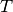such that:whereis the given flux,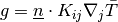, and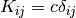(an isotropic medium). See the tutorial section Strong form of Poisson’s equation and its integration for a detailed explanation.

The diffusion velocity and fluxes through various parts of the boundary are computed in the post_process() function. On ‘Gamma_N’ (the Neumann condition boundary part), the flux/length should correspond to the given value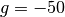, while on ‘Gamma_N0’ the flux should be zero. Use the ‘refinement_level’ option (see the usage examples below) to check the convergence of the numerical solution to those values. The total flux and the flux through ‘Gamma_D’ (the Dirichlet condition boundary part) are shown as well.

Usage Examples¶

Run with the default settings (no refinement):

python simple.py examples/diffusion/poisson_neumann.py


Refine the mesh twice:

python simple.py examples/diffusion/poisson_neumann.py -O "'refinement_level' : 2"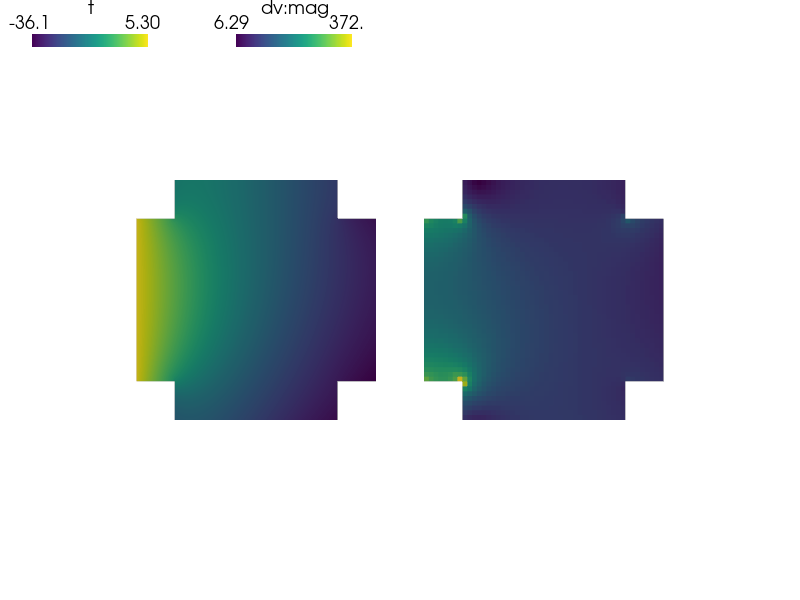source code

r"""
The Poisson equation with Neumann boundary conditions on a part of the
boundary.

Find :math:T such that:

.. math::
\int_{\Omega} K_{ij} \nabla_i s \nabla_j p T
= \int_{\Gamma_N} s g

where :math:g is the given flux, :math:g = \ul{n} \cdot K_{ij} \nabla_j
\bar{T}, and :math:K_{ij} = c \delta_{ij} (an isotropic medium). See the
tutorial section :ref:poisson-weak-form-tutorial for a detailed explanation.

The diffusion velocity and fluxes through various parts of the boundary are
computed in the :func:post_process() function. On 'Gamma_N' (the Neumann
condition boundary part), the flux/length should correspond to the given value
:math:g = -50, while on 'Gamma_N0' the flux should be zero. Use the
'refinement_level' option (see the usage examples below) to check the
convergence of the numerical solution to those values. The total flux and the
flux through 'Gamma_D' (the Dirichlet condition boundary part) are shown as
well.

Usage Examples
--------------

Run with the default settings (no refinement)::

python simple.py examples/diffusion/poisson_neumann.py

Refine the mesh twice::

python simple.py examples/diffusion/poisson_neumann.py -O "'refinement_level' : 2"
"""
from __future__ import absolute_import
import numpy as nm

from sfepy.base.base import output, Struct
from sfepy import data_dir

def post_process(out, pb, state, extend=False):
"""
Calculate :math:\nabla t and compute boundary fluxes.
"""
dv = pb.evaluate('ev_diffusion_velocity.i.Omega(m.K, t)', mode='el_avg',
verbose=False)
out['dv'] = Struct(name='output_data', mode='cell',
data=dv, dofs=None)

totals = nm.zeros(3)
for gamma in ['Gamma_N', 'Gamma_N0', 'Gamma_D']:

flux = pb.evaluate('d_surface_flux.i.%s(m.K, t)' % gamma,
verbose=False)
area = pb.evaluate('d_surface.i.%s(t)' % gamma, verbose=False)

flux_data = (gamma, flux, area, flux / area)
totals += flux_data[1:]

output('%8s flux: % 8.3f length: % 8.3f flux/length: % 8.3f'
% flux_data)

totals = totals / totals
output('   total flux: % 8.3f length: % 8.3f flux/length: % 8.3f'
% tuple(totals))

return out

filename_mesh = data_dir + '/meshes/2d/cross-51-0.34.mesh'

materials = {
'flux' : ({'val' : -50.0},),
'm' : ({'K' : 2.7 * nm.eye(2)},),
}

regions = {
'Omega' : 'all',
'Gamma_D' : ('vertices in (x < -0.4999)', 'facet'),
'Gamma_N0' : ('vertices in (y > 0.4999)', 'facet'),
'Gamma_N' : ('vertices of surface -s (r.Gamma_D +v r.Gamma_N0)',
'facet'),
}

fields = {
'temperature' : ('real', 1, 'Omega', 1),
}

variables = {
't' : ('unknown field', 'temperature', 0),
's' : ('test field',    'temperature', 't'),
}

ebcs = {
't1' : ('Gamma_D', {'t.0' : 5.3}),
}

integrals = {
'i' : 2
}

equations = {
'Temperature' : """
dw_diffusion.i.Omega(m.K, s, t)
= dw_surface_integrate.i.Gamma_N(flux.val, s)
"""
}

solvers = {
'ls' : ('ls.scipy_direct', {}),
'newton' : ('nls.newton', {
'i_max' : 1,
'eps_a' : 1e-10,
}),
}

options = {
'nls' : 'newton',
'ls' : 'ls',

'refinement_level' : 0,
'post_process_hook' : 'post_process',
}